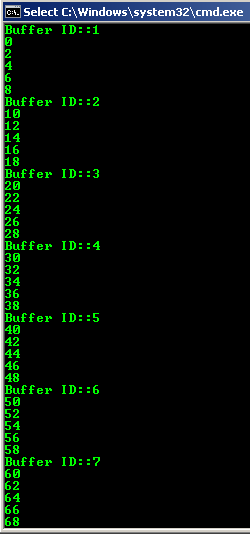## Friday, 4 May 2018

### Python: Functions - Part 1

Quick post Python functions:

Define a function and call it:

```def add(first_num, second_num):
sum = first_num + second_num
print(sum)

3
```

Two types of arguments: positional and keyword:
Positional - defined by order of parameters in the method
Keyword - use the parameter name when calling the function

You can forget what order to use when all of your arguments are keyword arguments:
```add(second_num = 3, first_num = 1)
```

But when mixing keyword arguments with positional you need to take care that all keyword arguments are at the end:

```add(second_num = 3, 3)
SyntaxError: positional argument follows keyword argument
```

In the call below, the first argument of value "1" is taken in as parameter "first_num" based on it's position, so defining it again as keyword Python will give error:

```>>> add(1, first_num = 3)
Traceback (most recent call last):
File "<pyshell#9>", line 1, in <module>
TypeError: add() got multiple values for argument 'first_num'
```

```>>> add(2, second_num = 3)
5
```

Default value:
Set a default value for a parameter in the method definition by using "=" sign.
In this example, the second_num parameter defaults to value of 5.
You can skip passing in second argument and the function will run fine.

```>>> def add(first_num, second_num = 5):
sum = first_num + second_num
print(sum)

8
```

If you don't want to use default value of 5, then call the function the usual way.

```>>> add(4, 5)
9
```

If you have many default parameters, you can use keyword to assign a different value to one of them and skip the rest. For example:

```>>> def calc(a, b, c = 10, d = 20, e = 30):
print(a + b + c + d + e)

# skip all default parameters
>>> calc(1, 2)
63

#when not using default val of 'e'
>>> calc(2, 3, e = 45)
80
```

Next post: Working with unknown number of parameters